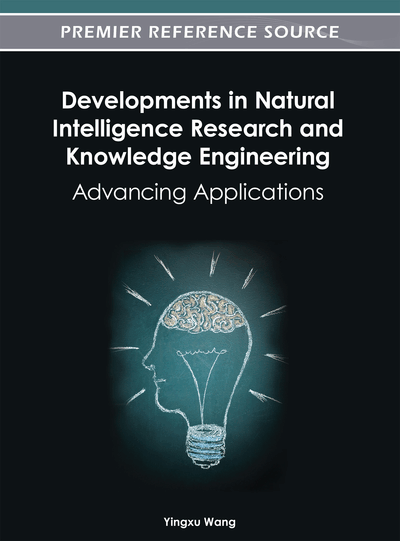# Further Considerations of Classification-Oriented and Approximation-Oriented Rough Sets in Generalized Settings

Masahiro Inuiguchi (Osaka University, Japan)
DOI: 10.4018/978-1-4666-1743-8.ch012

## Abstract

Rough sets can be interpreted in two ways: classification of objects and approximation of a set. From this point of view, classification-oriented and approximation-oriented rough sets have been proposed. In this paper, the author reconsiders those two kinds of rough sets with reviewing their definitions, properties and relations. The author describes that rough sets based on positive and negative extensive relations are mathematically equivalent but it is important to consider both because they obtained positive and negative extensive relations are not always in inverse relation in the real world. The difference in size of granules between union-based and intersection-based approximations is emphasized. Moreover, the types of decision rules associated with those rough sets are shown.
Chapter Preview
Top

## Introduction

The usefulness and efficiency of rough sets in analyses of data, information, decision and conflict have been demonstrated in the literatures (Lin, 1989a; Pawlak, 1991; Alpigini et al., 2002; Inuiguchi et al., 2003a; Wang et al., 2003). Rough set approaches have been developed mainly under equivalence relations. In order to enhance the ability of the analysis, as well as from the mathematical interests, rough sets have been generalized by many researchers (for example, (Lin, 1989a; Lin, 1989b; Dubois et al., 1990; Dubois et al., 1992; Lin, 1992; Ziarko, 1993; Yao et al., 1996; Bonikowski, 1998; Greco et al., 1999; Słowiński et al., 2000; Greco et al., 2003; Inuiguchi et al., 2003b; Inuiguchi et al., 2003c)). Among listed references, Ziarko (1993) generalized rough sets by parameterizing the accuracy while the others generalized rough sets by extending the equivalence relation of approximation space. In this paper, we concentrate on the latter generalizations.

Rough sets are often applied to analysis of decision tables, a collection of examples about object classifications by means of condition attributes. Considering classical rough set approaches to analysis of decision tables, the equivalence relation induced from the equality of condition attribute values implies that attributes are all nominal. Because of this assumption, unreasonable results for human intuition have been exemplified when some attributes are ordinal (Greco et al., 1999). To overcome the unreasonableness caused by the ordinal property, the dominance-based rough set approach has been proposed by Greco et al. (1999). Moreover, when the decision table includes missing values, the classical rough set approach does not work sufficiently. The generalization of rough sets is an interesting topic not only in practical point of view but also in mathematical point of view. Along this direction, rough sets have been generalized under neighborhood systems (Lin, 1989a; Lin, 1989b; Lin, 1992), similarity relations (Słowiński et al., 2000; Inuiguchi et al., 2003b), covers (Bonikowski, 1998; Inuiguchi et al., 2003b) and general relations (Yao et al., 1996) .The neighborhood systems (Lin, 1989a; Lin, 1989b; Lin, 1992) are the most general case that includes all these generalizations. Those results demonstrate a very far reaching generalization.

## Complete Chapter List

Search this Book:
Reset